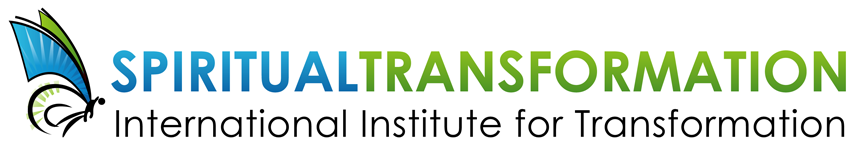# SEPTEMBER 2018

## Extra help with your spiritual journey this month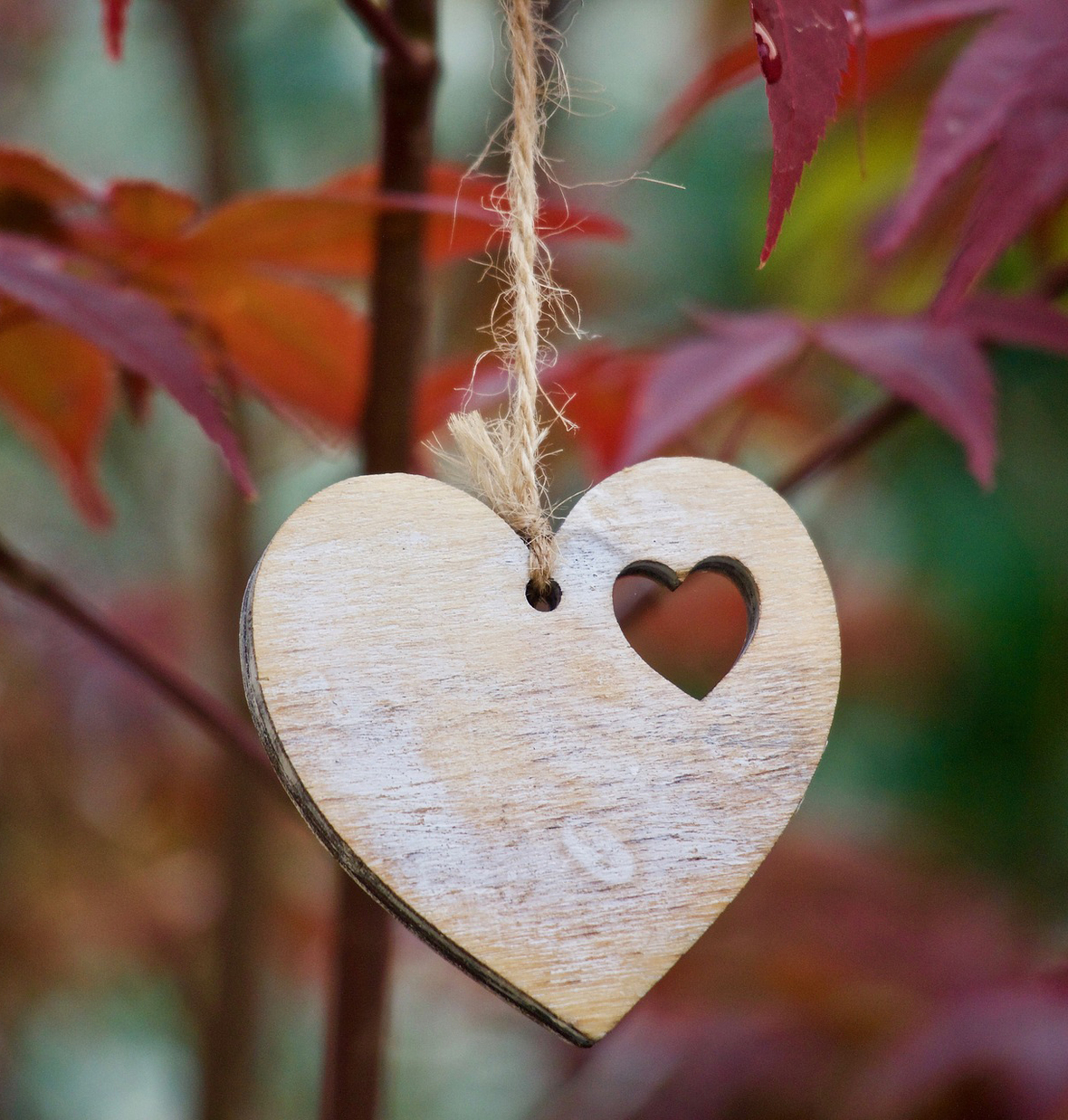Dear Friend,

There are so many ways that we can all be working on our spiritual transformation this fall! Here are some ways that IIT can help you:

▪ A brand new video of my talk on the "7 Kinds of Power" that we can have
▪ A 10% discount on my book, Manifest Your Soul's Purpose
▪ A 15% discount on all of our e-courses, newly revised and updated for 2018
▪ North American workshops on how to work with elementals and on one of my favourite topics, Hybrids
 ▪ A brand new video of my talk on the "7 Kinds of Power" that we can have
 ▪ A 10% discount on my book, Manifest Your Soul's Purpose
 ▪ A 15% discount on all of our e-courses, newly revised and updated for 2018
 ▪ North American workshops on how to work with elementals and on one of my favourite topics, Hybrids

Whew! There is sure to be something here to assist you on your journey.

Hugs from Tanis

 table div table+table+table+table+table div table{width:100%;padding:0}table div table+table+table+table+table div table img{width:96.23%;padding:0;float:none}table div table+table+table+table+table div table td{width:100%;padding:0 1.88% 18px}/* styles *//* styles */
 table div table+table+table+table+table+table+table div table{width:100%;padding:0}table div table+table+table+table+table+table+table div table img{width:96.23%;padding:0;float:none}table div table+table+table+table+table+table+table div table td{width:100%;padding:0 1.88% 18px}/* styles */## NEW VIDEO: 7 Kinds of Power

 /* styles */ In this new video, I talk about the various kinds of power we can have. Recorded in Freiburg, Germany in May 2018. In English with simultaneous translation into German. Enjoy this new video here: https://youtu.be/BK1EWdR_OkA
 table div table+table+table+table+table+table+table+table+table+table div table td,table.module-9{width:100%;padding:0}table div table+table+table+table+table+table+table+table+table+table div table{width:100%;float:none;margin-left:auto;margin-right:auto;padding:0}table div table+table+table+table+table+table+table+table+table+table div table a{border:0 none;text-decoration:none}table div table+table+table+table+table+table+table+table+table+table div table img{width:100%!important;border:0 none;text-decoration:none}/* styles */
 table div table+table+table+table+table+table+table+table+table+table+table div table{width:100%;padding:0}table div table+table+table+table+table+table+table+table+table+table+table div table img{width:96.23%;padding:0;float:none}table div table+table+table+table+table+table+table+table+table+table+table div table td{width:100%;padding:0 1.88% 18px}/* styles */# 10% off for the month of September!

Live your highest potential. Tanis Helliwell guides you in seeing how to apply spiritual truths in your everyday life. This book helps to develop you SQ-Spiritual Intelligence, PLUS:

▪ Reprogram Yourself for Success
▪ Master the Material World
▪ Cultivate People who Feed Your Soul
▪ Live in Harmony with Natural Cycles
▪ Walk the Path of Heart
 ▪ Reprogram Yourself for Success
 ▪ Master the Material World
 ▪ Cultivate People who Feed Your Soul
 ▪ Live in Harmony with Natural Cycles
 ▪ Walk the Path of Heart
 table div table+table+table+table+table+table+table+table+table+table+table+table+table+table+table div table{width:100%;padding:0}table div table+table+table+table+table+table+table+table+table+table+table+table+table+table+table div table img{width:96.23%;padding:0;float:none}table div table+table+table+table+table+table+table+table+table+table+table+table+table+table+table div table td{width:100%;padding:0 1.88% 18px}/* styles */## Fall 2018: Courses in North America & Europe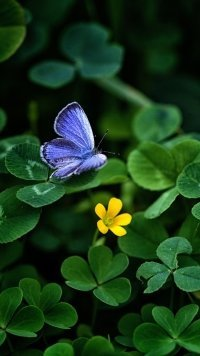# LAST CALL:

SEPTEMBER 29 -30, 2018ELEMENTALS AND CONSCIOUSNESS IN NATURE
This course will share with you the secrets of manifestation according to natural laws and the natural world so that you can be more effective in your work and world.

▪ Learn about leprechauns, fairies, elves, and meet one that wants to work with you.
▪ Meet your body elemental and work with it to heal your body.
▪ Learn about elementals and nature spirits of different countries and cultures.
▪ Create a beautiful world in co-operation with nature spirits.
▪ Weather permitting, some of the workshop will be held outdoors.
 ▪ Learn about leprechauns, fairies, elves, and meet one that wants to work with you.
 ▪ Meet your body elemental and work with it to heal your body.
 ▪ Learn about elementals and nature spirits of different countries and cultures.
 ▪ Create a beautiful world in co-operation with nature spirits.
 ▪ Weather permitting, some of the workshop will be held outdoors.

Contact: Tricia Tsybersma, tsybersma(at)hotmail.com, 1 (705) 446-8679
Price: \$150 per day or \$275 for two days includes snacks, light lunch
Accommodation: \$80 single, \$50 sharing includes breakfast

 table div table+table+table+table+table+table+table+table+table+table+table+table+table+table+table+table+table+table+table div table{width:100%;padding:0}table div table+table+table+table+table+table+table+table+table+table+table+table+table+table+table+table+table+table+table div table img{width:96.23%;padding:0;float:none}table div table+table+table+table+table+table+table+table+table+table+table+table+table+table+table+table+table+table+table div table td{width:100%;padding:0 1.88% 18px}/* styles */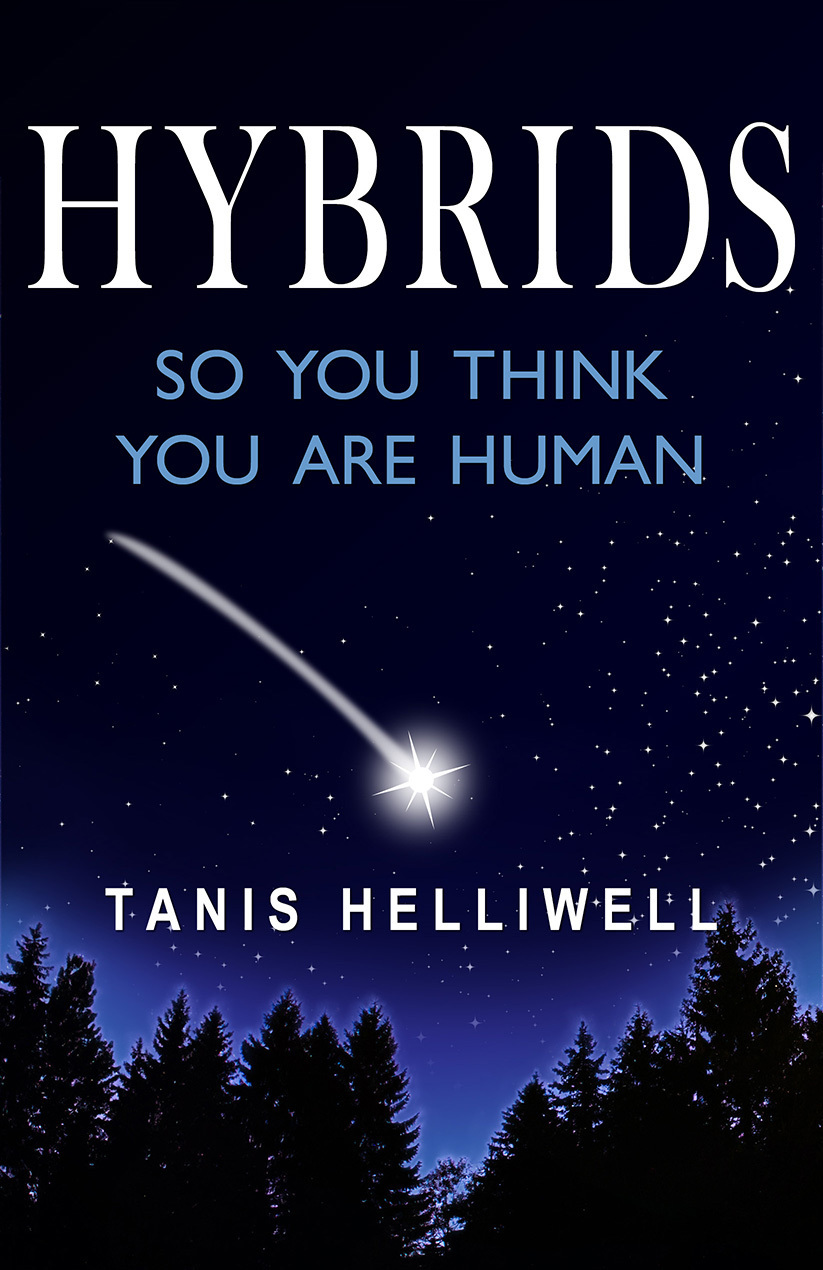OCTOBER 13-14, 2018HYBRIDS: SO YOU THINK YOU ARE HUMAN
Each of us is as unique as a snowflake and many of us are hybrids originally from a non-human evolution. Elementals, angels, dragons, bird beings, els, satyrs, beings from the Inner Earth are only a few of these evolutions.

▪ Discover your original evolution and therefore your purpose in this life
▪ Learn about the various kinds of hybrids and the characteristics of each
▪ How to recognize a hybrid from a human
▪ Ways to raise a hybrid child
▪ Mixed marriages between hybrids and humans
▪ How hybrids enrich our planet
 ▪ Discover your original evolution and therefore your purpose in this life
 ▪ Learn about the various kinds of hybrids and the characteristics of each
 ▪ How to recognize a hybrid from a human
 ▪ Ways to raise a hybrid child
 ▪ Mixed marriages between hybrids and humans
 ▪ How hybrids enrich our planet

Am I a Hybrid?
If you answer “yes” to these statements, Hybrids: So you think you are human will be of special interest to you:

▪ I often feel I don’t fit in
▪ I am hypersensitive to violence
▪ I am deeply committed to helping the Earth
▪ I would describe myself as an independent thinker
▪ I follow my own inner guidance about rightness, even if it goes against the norm
 ▪ I often feel I don’t fit in
 ▪ I am hypersensitive to violence
 ▪ I am deeply committed to helping the Earth
 ▪ I would describe myself as an independent thinker
 ▪ I follow my own inner guidance about rightness, even if it goes against the norm

Location: Institut de la conscience Eveilier, 336 Rue Robert, Ange-Garden, near Montreal, Canada
Contact: Sonya Roy info(at)sonyaroy.com 1 (778) 808-6101/ or 1 (450) 210-3101
Price: \$300

 table div table+table+table+table+table+table+table+table+table+table+table+table+table+table+table+table+table+table+table+table+table+table div table{width:100%;padding:0}table div table+table+table+table+table+table+table+table+table+table+table+table+table+table+table+table+table+table+table+table+table+table div table img{width:96.23%;padding:0;float:none}table div table+table+table+table+table+table+table+table+table+table+table+table+table+table+table+table+table+table+table+table+table+table div table td{width:100%;padding:0 1.88% 18px}/* styles */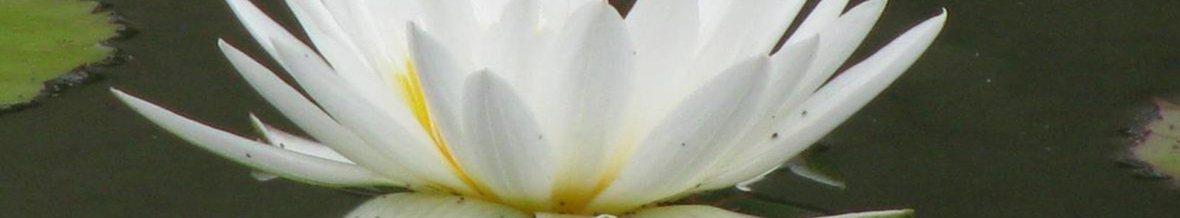## 15% off ALL e-Courses for September!

We have spent the last few months revising and improving all of our self study e-courses - and what a big job it's been!

To celebrate, we're offering a 15% discount on all of these courses for September only. Get your copies today and join or start a study group in your area. Fall 2018 study groups are already forming! Support each other on your spiritual transformation journey by joining an e-course self-study group. Contact study group facilitators here:

▪ North America, https://www.iitransform.com/north-america/
▪ Europe, https://www.iitransform.com/europe-2018/
 ▪ North America, https://www.iitransform.com/north-america/
 ▪ Europe, https://www.iitransform.com/europe-2018/

## Our e-courses are an excellent way to work an affordable spiritual retreat into your busy life!

 table div table+table+table+table+table+table+table+table+table+table+table+table+table+table+table+table+table+table+table+table+table+table+table+table+table+table div table{width:100%;padding:0}table div table+table+table+table+table+table+table+table+table+table+table+table+table+table+table+table+table+table+table+table+table+table+table+table+table+table div table img{width:96.23%;padding:0;float:none}table div table+table+table+table+table+table+table+table+table+table+table+table+table+table+table+table+table+table+table+table+table+table+table+table+table+table div table td{width:100%;padding:0 1.88% 18px}/* styles */# Transform Yourself (TYS) - 15% off

Has a life change got you looking for a life-changing experience?
Are you struggling with your purpose or mission in life?

Perhaps things are changing and you want or need a new perspective. Maybe you’re feeling a little out of control, down right afraid, or even immobilized. Has life got you considering a path that may not align with your real purpose?

Transform Yourself accelerates your spiritual transformation using the evolutionary new Etheric Clearing Technique (ECT). Clear away old thought patterns and align your life to your true purpose.

 table div table+table+table+table+table+table+table+table+table+table+table+table+table+table+table+table+table+table+table+table+table+table+table+table+table+table+table+table+table div table{width:100%;padding:0}table div table+table+table+table+table+table+table+table+table+table+table+table+table+table+table+table+table+table+table+table+table+table+table+table+table+table+table+table+table div table img{width:96.23%;padding:0;float:none}table div table+table+table+table+table+table+table+table+table+table+table+table+table+table+table+table+table+table+table+table+table+table+table+table+table+table+table+table+table div table td{width:100%;padding:0 1.88% 18px}/* styles */# Transform Your Work (TYW) - 15% off

Are you working to live or living to work?
* Are you looking for more fulfillment or meaning at work?

If your work-a-day world is draining your energy then it’s time to get an energy adjustment. The Transform Your Work self-study ecourse is designed to inspire your life and get you back in the flow.

 table div table+table+table+table+table+table+table+table+table+table+table+table+table+table+table+table+table+table+table+table+table+table+table+table+table+table+table+table+table+table+table+table div table{width:100%;padding:0}table div table+table+table+table+table+table+table+table+table+table+table+table+table+table+table+table+table+table+table+table+table+table+table+table+table+table+table+table+table+table+table+table div table img{width:96.23%;padding:0;float:none}table div table+table+table+table+table+table+table+table+table+table+table+table+table+table+table+table+table+table+table+table+table+table+table+table+table+table+table+table+table+table+table+table div table td{width:100%;padding:0 1.88% 18px}/* styles */# Spiritual Transformation (ST) - 15% off

Are you feeling restless or dissatisfied?
Are you tired of the struggle?
* Are blame and shame ruling your inner and outer world?

It might be time to give yourself a new perspective. You may be going through a spiritual transformation.

Recognize the signs of spiritual transformation, and unite your personality with your soul’s purpose giving you greater meaning in your life.

 table div table+table+table+table+table+table+table+table+table+table+table+table+table+table+table+table+table+table+table+table+table+table+table+table+table+table+table+table+table+table+table+table+table+table+table div table{width:100%;padding:0}table div table+table+table+table+table+table+table+table+table+table+table+table+table+table+table+table+table+table+table+table+table+table+table+table+table+table+table+table+table+table+table+table+table+table+table div table img{width:96.23%;padding:0;float:none}table div table+table+table+table+table+table+table+table+table+table+table+table+table+table+table+table+table+table+table+table+table+table+table+table+table+table+table+table+table+table+table+table+table+table+table div table td{width:100%;padding:0 1.88% 18px}/* styles */# Fear Transformed (FT) - 15% off

Are your fears holding you back?
Are you a slave to the negative effects of your past and worries about the future?
* Is fear controlling your life?

Sign up for the challenges that life presents – build an authentic brave heart!

Fear Transformed, is our newest self-study ecourse! This powerful new program will move you from fear to love.

 table div table+table+table+table+table+table+table+table+table+table+table+table+table+table+table+table+table+table+table+table+table+table+table+table+table+table+table+table+table+table+table+table+table+table+table+table+table+table div table{width:100%;padding:0}table div table+table+table+table+table+table+table+table+table+table+table+table+table+table+table+table+table+table+table+table+table+table+table+table+table+table+table+table+table+table+table+table+table+table+table+table+table+table div table img{width:96.23%;padding:0;float:none}table div table+table+table+table+table+table+table+table+table+table+table+table+table+table+table+table+table+table+table+table+table+table+table+table+table+table+table+table+table+table+table+table+table+table+table+table+table+table div table td{width:100%;padding:0 1.88% 18px}/* styles */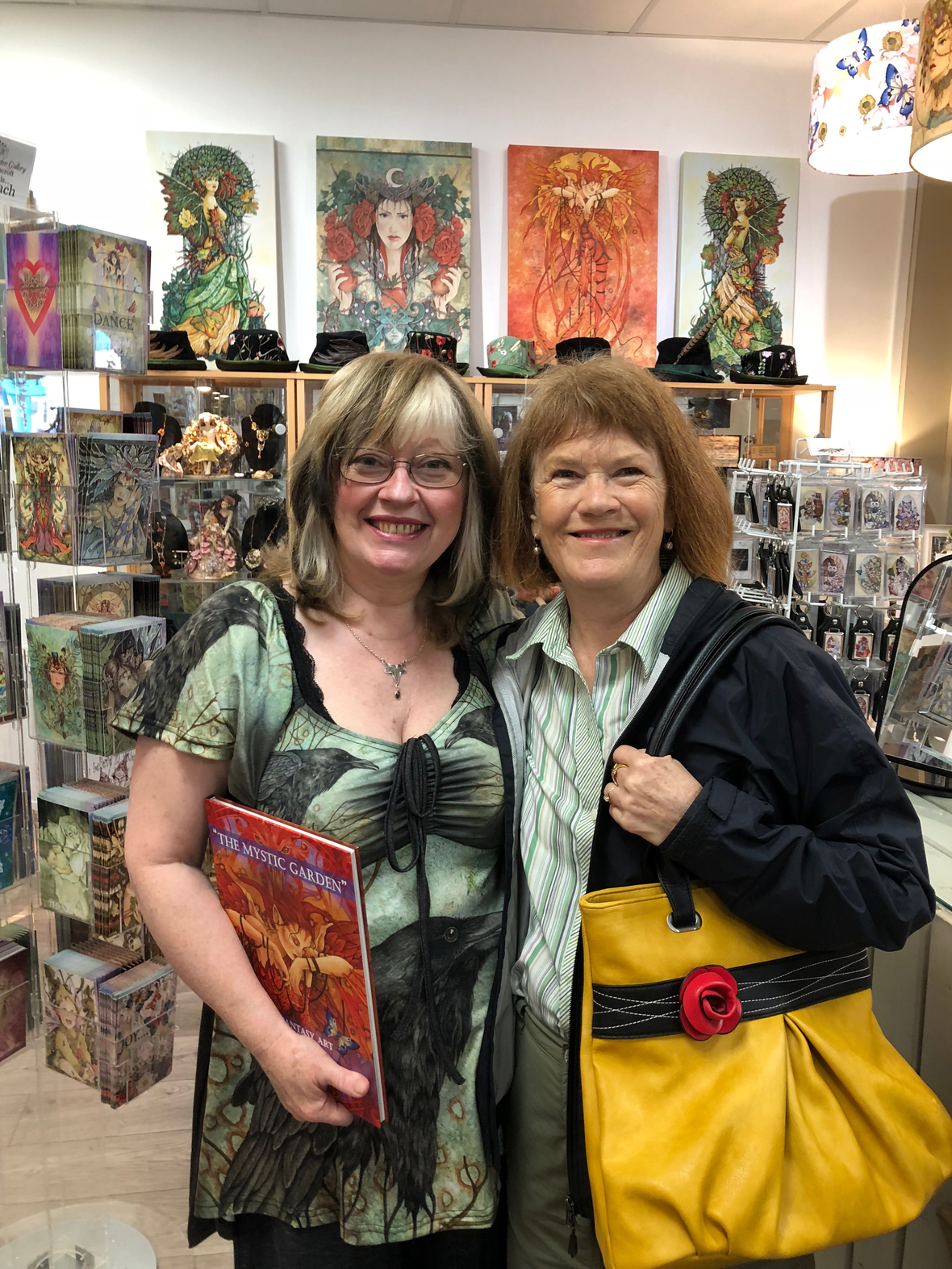Well-loved fairy painter Linda Ravenscroft and Tanis.
 table div table+table+table+table+table+table+table+table+table+table+table+table+table+table+table+table+table+table+table+table+table+table+table+table+table+table+table+table+table+table+table+table+table+table+table+table+table+table+table+table+table+table div table{width:100%;padding:0}table div table+table+table+table+table+table+table+table+table+table+table+table+table+table+table+table+table+table+table+table+table+table+table+table+table+table+table+table+table+table+table+table+table+table+table+table+table+table+table+table+table+table div table img{width:96.23%;padding:0;float:none}table div table+table+table+table+table+table+table+table+table+table+table+table+table+table+table+table+table+table+table+table+table+table+table+table+table+table+table+table+table+table+table+table+table+table+table+table+table+table+table+table+table+table div table td{width:100%;padding:0 1.88% 18px}/* styles */